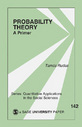Little Green Books

# Probability Theory

This book intends to give a non-technical introduction to probability theory, as it is used in the social sciences. The topics covered include the concept of probability and its relation to relative frequency, the properties of probability, discrete and continuous random variables, and binomial, uniform, normal and chi-squared distributions. Readers who have taken basic college mathematics will be comfortable with this work, which frequently draws intuition and examples instead of technically involved arguments to make its points. In spite of the elementary level of discussion, the concepts of continuous random variables and distributions are carefully developed. Thus, the book prepares the reader not only for a precise understanding of sampling theory, where discrete probabilities are used, but also to a deeper understanding of most of the statistical techniques applied in social science data analysis, where continuous probability distributions are often referenced.

locked icon

•••